JEE  >  Test: Continuity And Differentiability - 2

# Test: Continuity And Differentiability - 2

Test Description

## 25 Questions MCQ Test Mathematics (Maths) Class 12 | Test: Continuity And Differentiability - 2

Test: Continuity And Differentiability - 2 for JEE 2023 is part of Mathematics (Maths) Class 12 preparation. The Test: Continuity And Differentiability - 2 questions and answers have been prepared according to the JEE exam syllabus.The Test: Continuity And Differentiability - 2 MCQs are made for JEE 2023 Exam. Find important definitions, questions, notes, meanings, examples, exercises, MCQs and online tests for Test: Continuity And Differentiability - 2 below.
Solutions of Test: Continuity And Differentiability - 2 questions in English are available as part of our Mathematics (Maths) Class 12 for JEE & Test: Continuity And Differentiability - 2 solutions in Hindi for Mathematics (Maths) Class 12 course. Download more important topics, notes, lectures and mock test series for JEE Exam by signing up for free. Attempt Test: Continuity And Differentiability - 2 | 25 questions in 25 minutes | Mock test for JEE preparation | Free important questions MCQ to study Mathematics (Maths) Class 12 for JEE Exam | Download free PDF with solutions
 1 Crore+ students have signed up on EduRev. Have you?
Test: Continuity And Differentiability - 2 - Question 1

###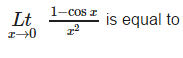Detailed Solution for Test: Continuity And Differentiability - 2 - Question 1

L'Hopital's rules says that the
lim x→a f(x)/g(x)
⇒ f'(a)/g'(a)
Using this, we get
lim x→0 (1−cosx)/x2
⇒ − sin0/2(0)
Yet as the denominator is 0, this is impossible. So we do a second limit:
lim(x→0) sinx/2x
⇒ cos0/2 = 1/2 = 0.5
So, in total lim x→0 (1−cosx)/x2
⇒ lim x→0 sinx/2x
⇒ cosx/2
⇒ cos0/2= 1/2

Test: Continuity And Differentiability - 2 - Question 2

### If f (x) is a polynomial of degree m (⩾1) , then which of the following is not true ?

Detailed Solution for Test: Continuity And Differentiability - 2 - Question 2

As all the three remaining statements are true for the given function.

Test: Continuity And Differentiability - 2 - Question 3

### Let f and g be differentiable functions such that fog = I, the identity function. If g’ (a) = 2 and g (a) = b, then f ‘ (b) =

Detailed Solution for Test: Continuity And Differentiability - 2 - Question 3

f(g(x)) = x
f'(g(x)) g'(x) = 1
put x = a
f'(b) g'(a) = 1
2 f'(b) = 1
f'(b) = 1/2

Test: Continuity And Differentiability - 2 - Question 4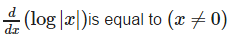Detailed Solution for Test: Continuity And Differentiability - 2 - Question 4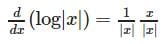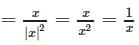Test: Continuity And Differentiability - 2 - Question 5

If f (x) =x2g(x) and g (x) is twice differentiable then f’’’ (x) is equal to

Detailed Solution for Test: Continuity And Differentiability - 2 - Question 5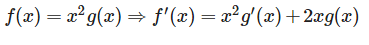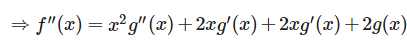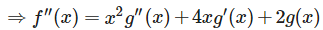Test: Continuity And Differentiability - 2 - Question 6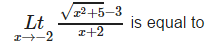Detailed Solution for Test: Continuity And Differentiability - 2 - Question 6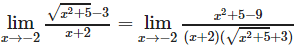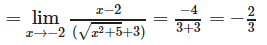Test: Continuity And Differentiability - 2 - Question 7

If f(x) = | x | ∀ x ∈ R, then

Detailed Solution for Test: Continuity And Differentiability - 2 - Question 7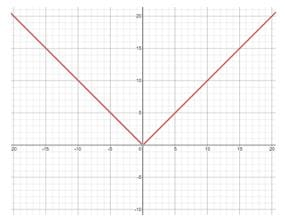The graph of f(x) = |x|
As observed from the graph, f(x) = |x| is continuous at x = 0.
As this curve is pointed at x = 0, f(x) is not derivable at x = 0.

Test: Continuity And Differentiability - 2 - Question 8

Differential coefficient of a function f (g (x)) w.r.t. the function g (x) is

Detailed Solution for Test: Continuity And Differentiability - 2 - Question 8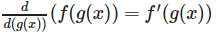Test: Continuity And Differentiability - 2 - Question 9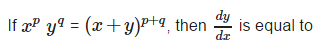Detailed Solution for Test: Continuity And Differentiability - 2 - Question 9

Given xpyq = (x+y)p+qTaking log on both sides we get:
plogx+qlogy = (p+q) log (x+y).  Differentiating both sides w.r.t. x we get ,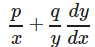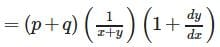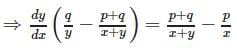Test: Continuity And Differentiability - 2 - Question 10

If y = aemx + be−mx, then y2 is equal to

Detailed Solution for Test: Continuity And Differentiability - 2 - Question 10

y = aemx + be-mx ⇒ y1 = amemx + (-m)be-mx ⇒y2

= am2emx + (m2)be-mx ⇒y2 = m2 (aemx + be-mx) ⇒ y2 = m2y

Test: Continuity And Differentiability - 2 - Question 11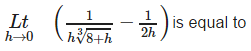Detailed Solution for Test: Continuity And Differentiability - 2 - Question 11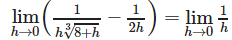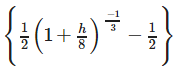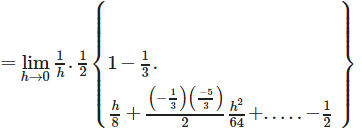Test: Continuity And Differentiability - 2 - Question 12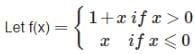then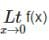is equal to

Test: Continuity And Differentiability - 2 - Question 13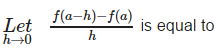Detailed Solution for Test: Continuity And Differentiability - 2 - Question 13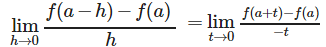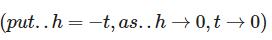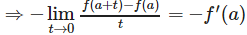Test: Continuity And Differentiability - 2 - Question 14

If f(x) be any function which assumes only positive values and f’ (x) exists then f’ (x) is equal to

Detailed Solution for Test: Continuity And Differentiability - 2 - Question 14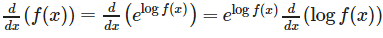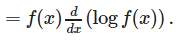Test: Continuity And Differentiability - 2 - Question 15

If y = a sin mx + b cos m x, then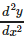is equal to

Detailed Solution for Test: Continuity And Differentiability - 2 - Question 15

y  = a sin mx+b cos mx ⇒ y1 = am cos mx − bm sin mx
⇒ y2 = −am2sin mx−bm2cosmx ⇒ y2 = −m2(a sin mx+b cos mx)

Test: Continuity And Differentiability - 2 - Question 16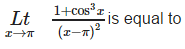Detailed Solution for Test: Continuity And Differentiability - 2 - Question 16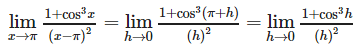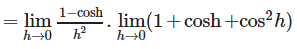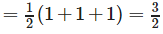Test: Continuity And Differentiability - 2 - Question 17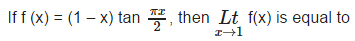Detailed Solution for Test: Continuity And Differentiability - 2 - Question 17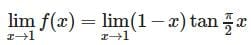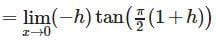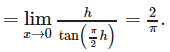Test: Continuity And Differentiability - 2 - Question 18

If y = tan−1x and z = cot−1x then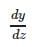is equal to

Detailed Solution for Test: Continuity And Differentiability - 2 - Question 18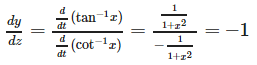Test: Continuity And Differentiability - 2 - Question 19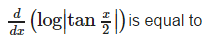Detailed Solution for Test: Continuity And Differentiability - 2 - Question 19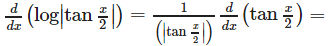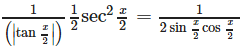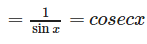Test: Continuity And Differentiability - 2 - Question 20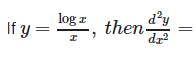Detailed Solution for Test: Continuity And Differentiability - 2 - Question 20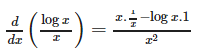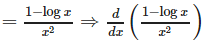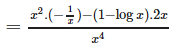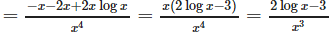Test: Continuity And Differentiability - 2 - Question 21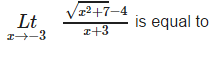Detailed Solution for Test: Continuity And Differentiability - 2 - Question 21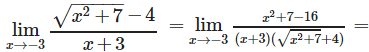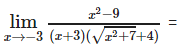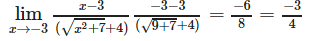Test: Continuity And Differentiability - 2 - Question 22

If both f and g are defined in a nhd of 0 ; f(0) = 0 = g(0) and f ‘ (0) = 8 = g’ (0), then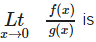equal to

Detailed Solution for Test: Continuity And Differentiability - 2 - Question 22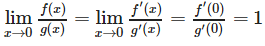(by using L’Hospital Rule)

Test: Continuity And Differentiability - 2 - Question 23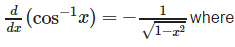Detailed Solution for Test: Continuity And Differentiability - 2 - Question 23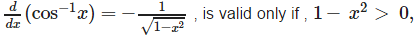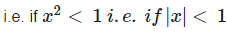Test: Continuity And Differentiability - 2 - Question 24

The differential coefficient of log (| log x |) w.r.t. log x is

Detailed Solution for Test: Continuity And Differentiability - 2 - Question 24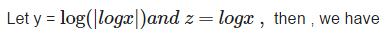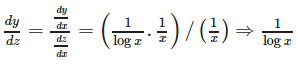Test: Continuity And Differentiability - 2 - Question 25

If f is derivable at x = a , then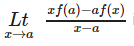is equal to

Detailed Solution for Test: Continuity And Differentiability - 2 - Question 25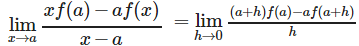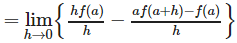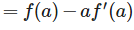## Mathematics (Maths) Class 12

209 videos|218 docs|139 tests
Information about Test: Continuity And Differentiability - 2 Page
In this test you can find the Exam questions for Test: Continuity And Differentiability - 2 solved & explained in the simplest way possible. Besides giving Questions and answers for Test: Continuity And Differentiability - 2, EduRev gives you an ample number of Online tests for practice

## Mathematics (Maths) Class 12

209 videos|218 docs|139 tests(Scan QR code)